Ch 4. Fundamental Laws (Integral Anal.) Multimedia Engineering Fluids ConservationMass LinearMomentum Moment ofMomentum ConservationEnergy
 Chapter 1. Basics 2. Fluid Statics 3. Kinematics 4. Laws (Integral) 5. Laws (Diff.) 6. Modeling/Similitude 7. Inviscid 8. Viscous 9. External Flow 10. Open-Channel Appendix Basic Math Units Basic Equations Water/Air Tables Sections Search eBooks Dynamics Fluids Math Mechanics Statics Thermodynamics Author(s): Chean Chin Ngo Kurt Gramoll ©Kurt GramollFLUID MECHANICS - CASE STUDY SOLUTION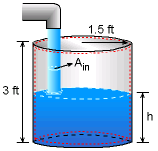Problem Diagram Take the entire tank as the control volume (red dashed lines). Applying the conservation of mass equation for this fixed and nondeforming control volume yields:The chosen control volume includes both air and water. Note that the rate of change of the mass of air inside the control volume should balance the mass of air flowing out, hence the conservation of mass can be written separately for air and water. For this problem, only the conservation of mass for water is needed. For water: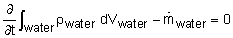The mass of water within the control volume is given by (assuming constant ρ)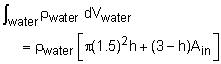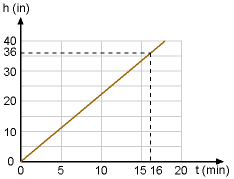Plot of Water Height as a Function of Time Hence, the continuity equation for the water becomes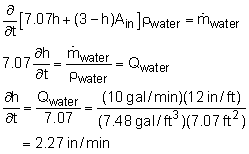Note that the cross sectional area of water flowing out of the faucet, Ain, is neglected since it is small compared to the cross sectional area of the tank. The total time needed to fill the empty tank is      t = 36 /2.27 = 16 min

Practice Homework and Test problems now available in the 'Eng Fluids' mobile app
Includes over 250 problems with complete detailed solutions.
Available now at the Google Play Store and Apple App Store.